# Week 7

## Case Studies

Lets look through some more examples that come with the CUDA SDK

here is a nice 30 minute webinar on global memory optimization in CUDA - http://developer.download.nvidia.com/CUDA/training/globalmemoryusage_june2011.mp4

and then back to OpenCL with some videos from the AMD Fusion Developer Summit from the summer of 2011

OpenCL and the 13 Dwarfs - 50 minute talk covering a lot of different uses for GPUs
video - http://developer.amd.com/afds/pages/OLD/video.aspx#/Dev_AFDS_Reb_2155
slides - http://developer.amd.com/afds/assets/presentations/2155_final.pdf

OpenCL with graphics using multiple graphics cards:
- video - http://developer.amd.com/afds/pages/OLD/video.aspx#/Dev_AFDS_Reb_2115
- slides - http://developer.amd.com/afds/assets/presentations/2115_final.pdf

Interesting talk on molecular docking that looks at the energy issues of using GPUs
- video - http://developer.amd.com/afds/pages/OLD/video.aspx#/Dev_AFDS_Reb_2130
- slides - http://developer.amd.com/afds/assets/presentations/2130_final.pdf

and here are some notes from a couple years ago looking at some more particle examples in CUDA

### This kind of thing is easier in CUDA. Here is an example derived from the CUDA OpenGL integration example which shows a simple particle system.

in this example each particle is generated and acts independently of the other particles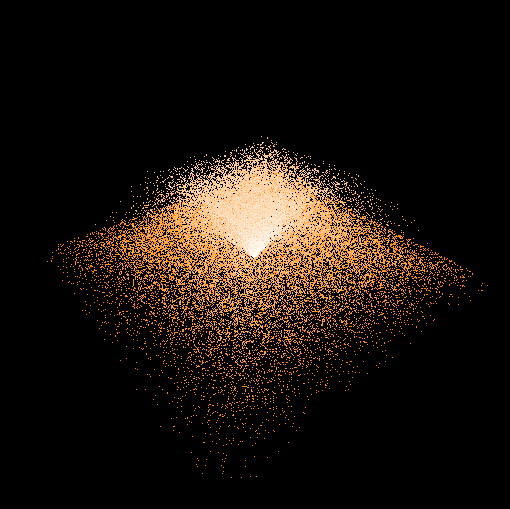__global__ void kernel(float4* pos, float4 * pdata, unsigned int width,
unsigned int height, int max_age, float time, float randy)
{
unsigned int x = blockIdx.x * blockDim.x + threadIdx.x;
unsigned int y = blockIdx.y * blockDim.y + threadIdx.y;
unsigned int arrayLoc = y*width + x;

float4 localData;
float4 localP;
float4 newOne;
float  cScale;

// if we are doing real work ...
if ((x < width) && (y < height))
{
// move the data we need into a register
localData = pdata[arrayLoc];
localP    = pos[arrayLoc];

// if the particle needs to re-spawn ...
if (localData.x >= max_age)
{
localData = make_float4(0.0,                                // age
0.02 * (x / (float) width - 0.5),   // horz velocity
0.015 + 0.01 * randy,               // vertical velocity
0.02 * (y / (float) height - 0.5)); // horz velocity

// move the generation point around
localP = make_float4(__sinf(time)*0.2,
0.0,
__cosf(time)*0.2,
0.0);
}

// take the current position and add on the velocity values
newOne = make_float4( localP.x + localData.y,
localP.y + localData.z,
localP.z + localData.w,
1.0f);

localData.x += 1.0;    // increase age
localData.z -= 0.0001; // feel the affects of gravity on vertical velocity

// does the particle hit the tabletop surface?
if ((newOne.y <= 0.0) && (localP.x*localP.x + localP.z*localP.z < 25.0))
localData.z *= -0.2;

// now need to modify the color info in the array based on age of particle
cScale =  localData.x / max_age;

// move the particle data back to global memory
pdata[arrayLoc] = localData;

// write out color (r, g, b, alpha) into the vbo
// pos[width*height] is where the colours start
pos[width*height + arrayLoc] = make_float4(1.0,
1.0 - 0.5 * cScale,
1.0 - cScale,
0.0);

// write output vertex x, y, z, w
pos[arrayLoc] = newOne;
}
}

void createVBO(GLuint* vbo)
{
// create buffer object
glGenBuffers( 1, vbo);
glBindBuffer( GL_ARRAY_BUFFER, *vbo);

// initialize buffer object
unsigned int size = mesh_width * mesh_height * 8 * sizeof( float);
glBufferData( GL_ARRAY_BUFFER, size, 0, GL_DYNAMIC_DRAW);

glBindBuffer( GL_ARRAY_BUFFER, 0);

// register buffer object with CUDA
CUDA_SAFE_CALL(cudaGLRegisterBufferObject(*vbo));

CUT_CHECK_ERROR_GL();
}

void runCuda( GLuint vbo)
{
// map OpenGL buffer object for writing from CUDA
float4 *dptr;
CUDA_SAFE_CALL(cudaGLMapBufferObject( (void**)&dptr, vbo));

// execute the kernel
dim3 block(16, 16, 1);
dim3 grid(mesh_width / block.x, mesh_height / block.y, 1);
kernel<<< grid, block>>>(dptr, d_particleData, mesh_width, mesh_height,
max_age, anim, (rand()%1000)/1000.0);

// here we have a mesh_width and mesh_height of 256
// each block contains an 16x16 array of kernels so the grid contains 16 x 16 blocks

// unmap buffer object
CUDA_SAFE_CALL(cudaGLUnmapBufferObject( vbo));
}

void display()
{
// run CUDA kernel to generate vertex positions
runCuda(vbo);

glClear(GL_COLOR_BUFFER_BIT | GL_DEPTH_BUFFER_BIT);

// set view matrix
glMatrixMode(GL_MODELVIEW);
glTranslatef(0.0, 0.0, translate_z);
glRotatef(rotate_x, 1.0, 0.0, 0.0);
glRotatef(rotate_y, 0.0, 1.0, 0.0);

// render from the vbo
glBindBuffer(GL_ARRAY_BUFFER, vbo);
glVertexPointer(4, GL_FLOAT, 0, 0); // size, type, stride, pointer
glColorPointer(4, GL_FLOAT, 0, (GLvoid *) (mesh_width * mesh_height *
sizeof(float)*4));

glEnableClientState(GL_VERTEX_ARRAY);
glEnableClientState(GL_COLOR_ARRAY);

glDrawArrays(GL_POINTS, 0, mesh_width * mesh_height);

glDisableClientState(GL_VERTEX_ARRAY);
glDisableClientState(GL_COLOR_ARRAY);

glutSwapBuffers();
glutPostRedisplay();

anim += 0.01;
}

// constants

const unsigned int mesh_width  = 256;
const unsigned int mesh_height = 256;

const unsigned int max_age     = 600;

// vbo variables
GLuint vbo;

float anim = 0.0;

float4 * d_particleData;
float4 * h_particleData;

void runTest( int argc, char** argv)
{
CUT_DEVICE_INIT(argc, argv);

// Create GL context
glutInit( &argc, argv);
glutInitDisplayMode( GLUT_RGBA | GLUT_DOUBLE);
glutInitWindowSize( window_width, window_height);
glutCreateWindow( "Cuda GL interop");

// initialize GL
if( CUTFalse == initGL()) {
return;
}

// register callbacks
glutDisplayFunc( display);
glutKeyboardFunc( keyboard);
glutMouseFunc( mouse);
glutMotionFunc( motion);

// create VBO - each element has 8 floats (x, y, z, w, r, g, b, alpha)
createVBO( &vbo);

// create a new array to hold the data on the particles on the host
// array has mesh_width x mesh_height elements
// age, x-velocity, y-velocity, z-velocity (each of them a float)

h_particleData = (float *) malloc (4 * mesh_width * mesh_height * sizeof(float));

// an a similar array on the device

CUDA_SAFE_CALL(cudaMalloc( (void**) &d_particleData,
4 * mesh_width * mesh_height * sizeof(float)));

// initialize the particles

int pCounter;
for (pCounter = 0; pCounter < mesh_width * mesh_height; pCounter ++)
{
// set age to a random age so particles will spawn over time
h_particleData[4*pCounter] = rand() % max_age; // age

// set all the velocities to get them off the screen
h_particleData[4*pCounter+1] = -10000;
h_particleData[4*pCounter+2] = -10000;
h_particleData[4*pCounter+3] = -10000;
}

// copy the particle data from the host over to the card

CUDA_SAFE_CALL(cudaMemcpy (d_particleData, h_particleData,
4 * mesh_width * mesh_height *
sizeof(float),
cudaMemcpyHostToDevice));

// run the cuda part
runCuda( vbo);

// start rendering mainloop
glutMainLoop();
}

in terms of how speed is affected by kernel block layout
256, 1 -> 75 fps
16, 16 -> 75 fps

16,  8 -> 75 fps
32, 16 -> 75 fps
32,  8 -> 75 fps

32,  4 -> 75 fps
8,  8 -> 73 fps
4,  4 -> 73 fps
8, 16 -> 72 fps
2,  2 -> 69 fps
1,256 -> 61 fps
1,  1 -> 49 fps

With a few modifications we can create a simple swarm where the location of the center of the swarm is passed into the kernel and all of the particles try to head towards the center.// for a change of pace this kernel has pdata as a flot * rather than a float4 *
__global__ void kernel(float4* pos, float * pdata, unsigned int width,
unsigned int height, int max_age, float time,
float randy1, float randy2, float randy3,
float tx, float ty, float tz)
{
unsigned int x = blockIdx.x*blockDim.x + threadIdx.x;
unsigned int y = blockIdx.y*blockDim.y + threadIdx.y;

unsigned int arrayLoc = y*width*4 + x*4;
unsigned int posLoc = y*width+x;

float rx, ry, rz;
float vx, vy, vz;
float dx, dy, dz, sum;

if (pdata[arrayLoc] >= max_age)
{
rx = (randy1 - 0.5);
ry = (randy2 - 0.5);
rz = (randy3 - 0.5);

pdata[arrayLoc] = 0;
pdata[arrayLoc+1] = 0.001 * rx * rx * rx;
pdata[arrayLoc+2] = 0.001 * ry * ry * ry;
pdata[arrayLoc+3] = 0.001 * rz * rz * rz;

// any new ones spawn near the target

pos[posLoc].x = tx + 2.0 * rx * rx * rx;
pos[posLoc].y = ty + 2.0 * ry * ry * ry;
pos[posLoc].z = tz + 2.0 * rz * rz * rz;
}

pdata[arrayLoc] += 1;        // increase age

dx = (tx - pos[posLoc].x);
dy = (ty - pos[posLoc].y);
dz = (tz - pos[posLoc].z);
sum = sqrt(dx*dx + dy*dy + dz*dz);

// update the velocity
vx = 0.000005 * dx/sum;
vy = 0.000005 * dy/sum;
vz = 0.000005 * dz/sum;

pdata[arrayLoc+1] = pdata[arrayLoc+1] + vx;
pdata[arrayLoc+2] = pdata[arrayLoc+2] + vy;
pdata[arrayLoc+3] = pdata[arrayLoc+3] + vz;

float newX = pos[posLoc].x + pdata[arrayLoc+1];
float newY = pos[posLoc].y + pdata[arrayLoc+2];
float newZ = pos[posLoc].z + pdata[arrayLoc+3];

// now need to modify the color info in the array

pos[width*height + posLoc].x = 1.0;
pos[width*height + posLoc].y = 1.0 - 0.5 * pdata[arrayLoc]/max_age;
pos[width*height + posLoc].z = 1.0 - pdata[arrayLoc]/max_age;

// write output vertex
pos[posLoc] = make_float4(newX, newY, newZ, 1.0f);
}

or the particles can move away from the center and fall like a sparklerAnother option is to have each particle try to move towards the center of the swarm in a very simplified version of gravity. Here each particle computes the distance and direction to all of the other particles to work out the sum of those 'forces.' In this case since the computation is much greater there are many fewer particles.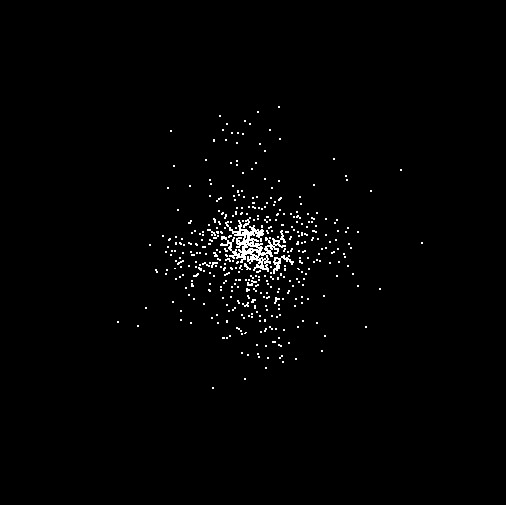Note that this code is most definitely not optimized.

__global__ void kernel(float4* pos, float4 * pdata, unsigned int width,
unsigned int height)
{
unsigned int x = blockIdx.x*blockDim.x + threadIdx.x;
unsigned int y = blockIdx.y*blockDim.y + threadIdx.y;

if ((x >= width) || (y >= height))
return;

unsigned int posLoc   = y*width+x;

int i;
float3 affect = {0.0, 0.0, 0.0};
float3 dif, p;
float3 newOne;

// in larger problems float4 might be better

for (i=0; i <width * height; i++)
{
dif.x = 1000 * (pos[i].x - pos[posLoc].x);
dif.y = 1000 * (pos[i].y - pos[posLoc].y);
dif.z = 1000 * (pos[i].z - pos[posLoc].z);

if (fabs(dif.x) > 0.1)
affect.x += (1/dif.x);

if (fabs(dif.y) > 0.1)
affect.y += (1/dif.y);

if (fabs(dif.z) > 0.1)
affect.z += (1/dif.z);
}

p = affect;
p.x += pdata[posLoc].y;
p.y += pdata[posLoc].z;
p.z += pdata[posLoc].w;

newOne.x = pos[posLoc].x + 0.0000001 * p.x;
newOne.y = pos[posLoc].y + 0.0000001 * p.y;
newOne.z = pos[posLoc].z + 0.0000001 * p.z;

// write out the current velocity information
pdata[posLoc] = make_float4(0, p.x, p.y, p.z);

// map r, g, b colour to x, y, z velocity
if (p.x > 0)
pos[width*height + posLoc].x = 1.0;
else
pos[width*height + posLoc].x = 0.4;

if (p.y > 0)
pos[width*height + posLoc].y = 1.0;
else
pos[width*height + posLoc].y = 0.4;

if (p.z > 0)
pos[width*height + posLoc].z = 1.0;
else
pos[width*height + posLoc].z = 0.4;

// write output vertex
pos[posLoc] = make_float4(newOne.x, newOne.y, newOne.z, 1.0f);
}

and if we take that further we can get the basis of the galaxy collision simulation the class did as their second project last time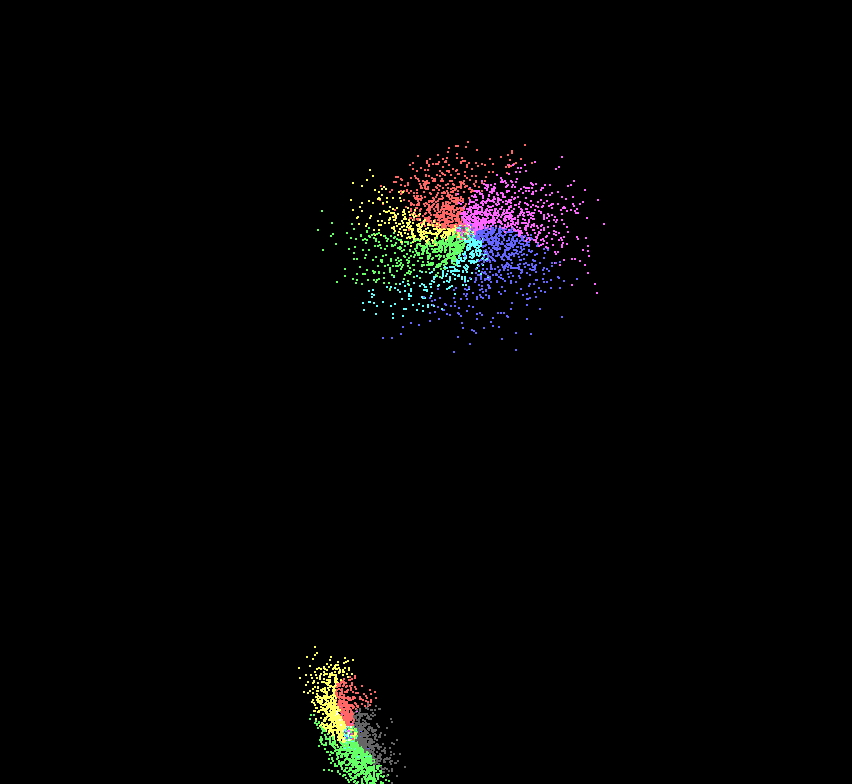### Optimization

We currently have a maximum of 512 threads per block.

Threads are assigned to streaming processors in blocks. Up to 8 blocks can be assigned to a single streaming multiprocessor ... BUT ... in the G80 card each streaming multiprocessor can run a max of 768 threads at once. To max out a streaming multiprocessor you could have 3 blocks each with 256 threads or 6 blocks with 128 threads.

A 'warp' is the scheduling unit for the streaming multiprocessor. On the G80 each block is executed in 32-thread warps - so the first 32 threads in the block are warp 1, the next 32 threads are warp 2, etc. If you have 2 blocks, each with 256 threads then there will be 8 warps generated per block (256 / 32 = 8) and so there will be 16 warps total.

Warps are not visible in the language - they may change radically in the future.

The CUDA occupancy calculator excel spreadsheet might be able to help: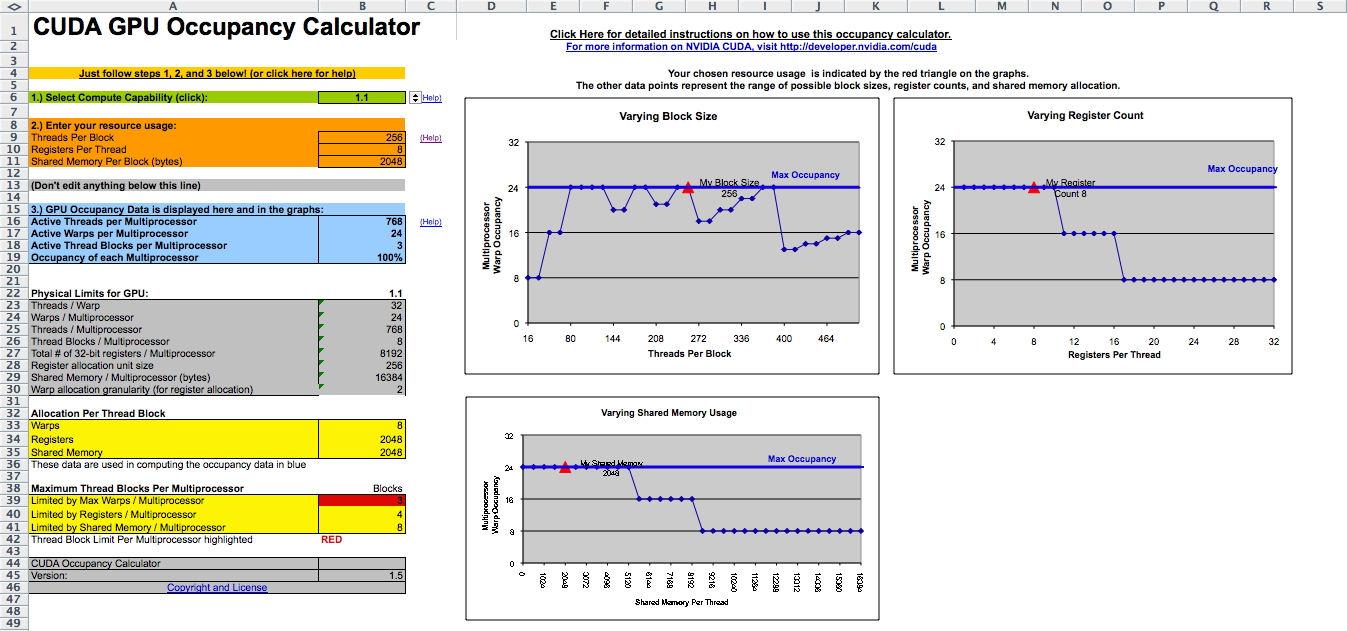There are lots of common functions available (but no rand) and there are also less accurate faster device only versions e.g. __sin, __pow, __log, __exp . You can use -use_fast_math to force all the math functions to use the faster low precision versions.

CUDA and graphics threads take turns on the GPU switching between kernels on the cuda side and between primitives on the graphics side.

The more threads that are running implies fewer registers available to each of those threads e.g. the 8800 has 8192 registers per block shared among all the threads in that block.

Variables declared without any qualifiers (shared, constant) will be allocated on a register unless there are no more registers in which case it will be allocated on local memory. Arrays will be allocated in local memory.

Global variables are stored in DRAM which is much slower than shared memory - so a good idea is to partition data into tiles that can fit into shared memory, have one thread block do the computation with that tile, and write the results back out to global memory

Constant variables are stored in DRAM, so it should be slow, but its cached so its very efficient for read-only data. Constant variables can only be assigned a value from the host

Shared variables can not be initialized as part of their declaration

So here are some simple rules:
data is read/write within a block -> shared
data is read/write inputs/results -> global

New cards will have double precision and those will be used by default which will be slower and you may not need the extra precision, so you should add an 'f' onto the end of literals, e.g. 3.14f vs 3.14 and onto the functions, e.g. sinf() instead of sin

Its important to avoid Bank Conflicts in shared memory as described in Section 5.1.2.5 on page 60 in version 2 of the CUDA Programming guide.

Shared memory is divided into equally-sized memory modules, called banks, which can be accessed simultaneously. So, any memory read or write request made of n addresses that fall in n distinct memory banks can be serviced simultaneously, yielding an effective bandwidth that is n times as high as the bandwidth of a single module.

However, if two addresses of a memory request fall in the same memory bank, there is a bank conflict and the access has to be serialized. The hardware splits a memory request with bank conflicts into as many separate conflict-free requests as necessary, decreasing the effective bandwidth by a factor equal to the number of separate memory requests.

In particular, warps are currently of size 32, and shared memory is divided into 16 banks where successive 32-bit words are assigned to successive banks. A shared memory request for a warp is split into one request
for the first half of the warp and one request for the second half of the warp so there can't be conflicts between those two half-warps. However within a half-warp all 16 threads could try to access shared memory within the same bank.

Pinning memory may also help by using cudaMallocHost() rather than malloc() if you have regular communication between the device and the host.

You should also be careful to minimize the movement of data between the host and the device.

Parts 4 and 5 of the Dr Dobbs article from last week discussed more about optimization:
http://www.ddj.com/hpc-high-performance-computing/207200659

and the CUDA C best practices guide:

My original particle code looked like this:

__global__ void kernel(float4* pos, float * pdata, unsigned int width,
unsigned int height, int max_age, float time, float randy)
{
unsigned int x = blockIdx.x*blockDim.x + threadIdx.x;
unsigned int y = blockIdx.y*blockDim.y + threadIdx.y;

unsigned int arrayLoc = y*width*4 + x*4;
unsigned int posLoc = y*width+x;

if (pdata[arrayLoc] >= max_age)
{
pdata[arrayLoc] = 0;
pdata[arrayLoc+1] = 0.02 * (x / (float) width - 0.5);
pdata[arrayLoc+2] = 0.015 + 0.01 * randy;
pdata[arrayLoc+3] = 0.02 * (y / (float) height - 0.5);

// maybe move the generation point around?

pos[posLoc].x = sin(time)/5.0;
pos[posLoc].y = 0;
pos[posLoc].z = cos(time)/5.0;
}

float newX = pos[posLoc].x + pdata[arrayLoc+1];
float newY = pos[posLoc].y + pdata[arrayLoc+2];
float newZ = pos[posLoc].z + pdata[arrayLoc+3];

pdata[arrayLoc] += 1;        // increase age
pdata[arrayLoc+2] -= 0.0001; // gravity

// tabletop surface
if ((newY <= 0) && fabs(pos[posLoc].x)<5 && fabs(pos[posLoc].z)<5)
{
pdata[arrayLoc+2] = -0.2 * pdata[arrayLoc+2];
}

// now need to modify the color info in the array
pos[width*height + posLoc].x = 1.0;
pos[width*height + posLoc].y = 1.0 - 0.5 * pdata[arrayLoc]/max_age;
pos[width*height + posLoc].z = 1.0 - pdata[arrayLoc]/max_age;

// write output vertex
pos[posLoc] = make_float4(newX, newY, newZ, 1.0f);
}

from above and tried to do some optimizing on it. First I did some performance testing using CUT_SAFE_CALL(cutCreateTimer(&timer)); and CUT_SAFE_CALL(cutStartTimer(timer)); and CUT_SAFE_CALL(cutStopTimer(timer));  and cutGetAverageTimerValue(timer) in the main program.

With a 256 x 256 VBO of particles and the code above I varied the arrangement of kernels in each block which influenced the arrangement of blocks in the grid and timed the application on both the 8600M GT and 9600 GT.

Then I tried to improve the kernel. The biggest improvement was in using an array of float4s instead of just floats for the pdata and adjusting how the kernels were assigned to the blocks. Lesser improvements involved reducing the number of reads from global memory by doing one read to copy those values into shared memory or local registers to do the computation there. I also tried adding some __syncthreads but those only had the affect of reducing performance by 1%. The faster sin and cos functions are taking up no time. I think I gain a little by replacing the fabs calls with multiplication and reducing one if statement.

 Blocks Grid 8600M GT fps 8600M GT * fps 9600 GT fps 9600 GT * fps 1 x 1 256 x 256 40 55 245 430 2 x 2 128 x 128 71 84 545 920 8 x 8 32 x 32 80 89 730 1100 12 x 12 22 x 22 80 88 710 1080 16 x 16 16 x 16 82 94 830 1350 20 x 20 13 x 13 78 90 690 1130 16 x 1 16 x 256 1360 1 x 16 256 x 1 1060 256 x 1 1 x 256 1330 1 x 256 256 x 1 625

in graphical form we can show the relative fps gains as follows where the blue bars show the naive version of the code and the green bars show the optimized version of the code. There is not a large improvement on the laptop card, but there is a significant improvement on the newer desktop card.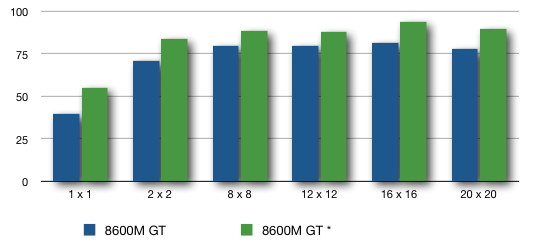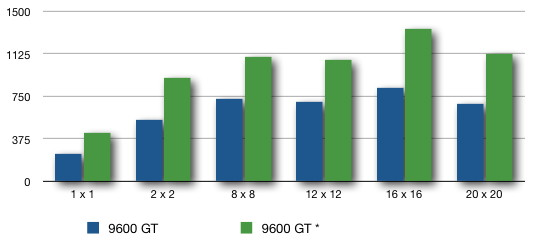I can push the frame rate for the 16 x 16 case on the 9600 GT up to 1515 by declaring a BLOCK_DIM by BLOCK_DIM region of float4 shared memory and copying either pdata or pos (but not both) into that shared memory at the beginning of the kernel. This increases to 1530 with updating that shared memory at the end of the kernel and then copying from shared memory back to global memory. Since we aren't doing that much work in shared memory registers should have a similar effect here.

From my original starting point at 730 fps I was able to boost performance to 1530 fps without too much work.

another tool worth looking at is the visual profiler

### Project 2 presentations from the class

last revision 2/29/12